## Free Statistics

of Irreproducible Research!

Author's title
Author*Unverified author*
R Software Modulerwasp_meanplot.wasp
Title produced by softwareMean Plot
Date of computationSun, 30 Dec 2012 09:14:54 -0500
Cite this page as followsStatistical Computations at FreeStatistics.org, Office for Research Development and Education, URL https://freestatistics.org/blog/index.php?v=date/2012/Dec/30/t1356877072fayzix6yarlz418.htm/, Retrieved Tue, 28 Nov 2023 23:38:43 +0000
Statistical Computations at FreeStatistics.org, Office for Research Development and Education, URL https://freestatistics.org/blog/index.php?pk=204950, Retrieved Tue, 28 Nov 2023 23:38:43 +0000
QR Codes:Original text written by user:
IsPrivate?No (this computation is public)
User-defined keywords
Estimated Impact113
Family? (F = Feedback message, R = changed R code, M = changed R Module, P = changed Parameters, D = changed Data)
-     [Mean Plot] [Mean plot eigen r...] [2012-12-27 11:32:26] [270271722390d2035805c3d10dfa13a8]
- R PD    [Mean Plot] [Mean plot eigen r...] [2012-12-30 14:14:54] [56be9a844975c6d0d36e88eaea5fb75b] [Current]
Feedback Forum

Post a new message
Dataseries X:
45.3
49.9
53.8
55.1
52.9
53.5
53.8
52
48.2
45.5
45.7
52.5
52.3
54.8
54.7
54.9
54.9
64.2
66.4
69.1
68.3
77.3
89.6
93
96.1
131.3
125.3
126
138.3
163
182.5
164.6
148.8
109.3
93.5
80.2
84
75.5
62.4
64.2
64.7
71
73.7
72.6
68.1
72.3
78.5
81.9
97.8
93.1
94.2
101.1
101
99.7
97.1
91.7
95
98.9
109
121.9
131.5
128.5
128.4
126.4
123.1
123
123.3
123.6
124.9
120.4
114.9
113.4

 Summary of computational transaction Raw Input view raw input (R code) Raw Output view raw output of R engine Computing time 5 seconds R Server 'Gwilym Jenkins' @ jenkins.wessa.net

\begin{tabular}{lllllllll}
\hline
Summary of computational transaction \tabularnewline
Raw Input & view raw input (R code)  \tabularnewline
Raw Output & view raw output of R engine  \tabularnewline
Computing time & 5 seconds \tabularnewline
R Server & 'Gwilym Jenkins' @ jenkins.wessa.net \tabularnewline
\hline
\end{tabular}
%Source: https://freestatistics.org/blog/index.php?pk=204950&T=0

[TABLE]
[ROW][C]Summary of computational transaction[/C][/ROW]
[ROW][C]Raw Input[/C][C]view raw input (R code) [/C][/ROW]
[ROW][C]Raw Output[/C][C]view raw output of R engine [/C][/ROW]
[ROW][C]Computing time[/C][C]5 seconds[/C][/ROW]
[ROW][C]R Server[/C][C]'Gwilym Jenkins' @ jenkins.wessa.net[/C][/ROW]
[/TABLE]
Source: https://freestatistics.org/blog/index.php?pk=204950&T=0

Globally Unique Identifier (entire table): ba.freestatistics.org/blog/index.php?pk=204950&T=0

As an alternative you can also use a QR Code:

The GUIDs for individual cells are displayed in the table below:

 Summary of computational transaction Raw Input view raw input (R code) Raw Output view raw output of R engine Computing time 5 seconds R Server 'Gwilym Jenkins' @ jenkins.wessa.net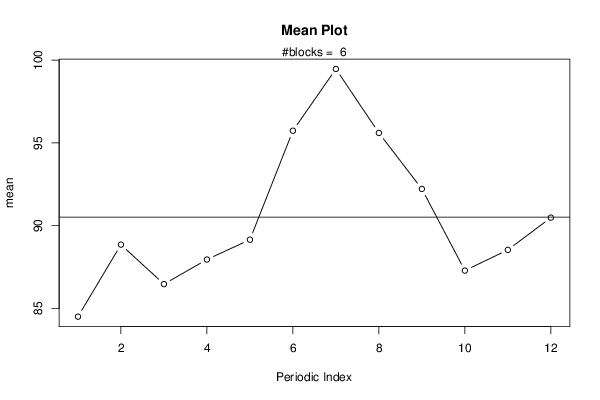PNG link Postscript link PDF link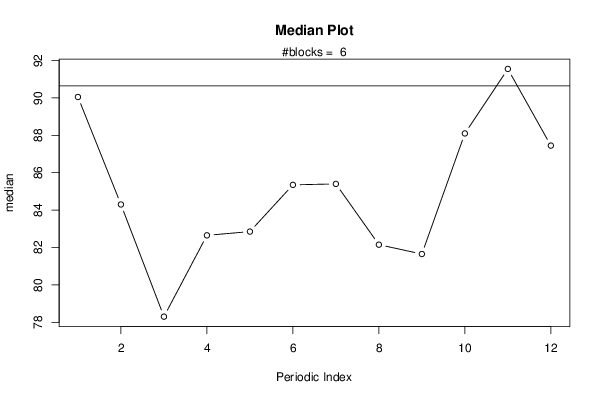PNG link Postscript link PDF link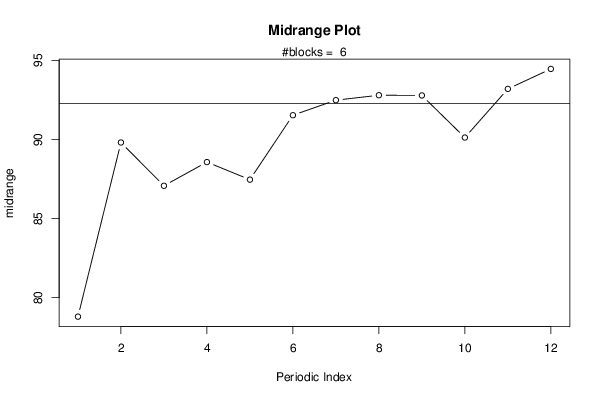PNG link Postscript link PDF link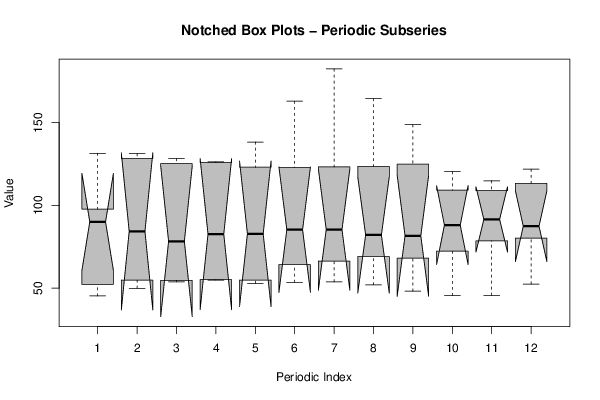PNG link Postscript link PDF link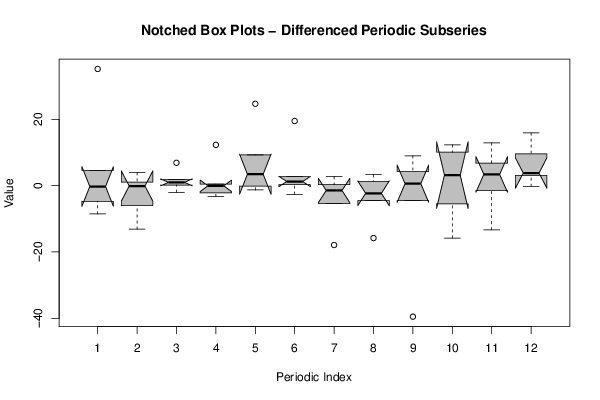PNG link Postscript link PDF link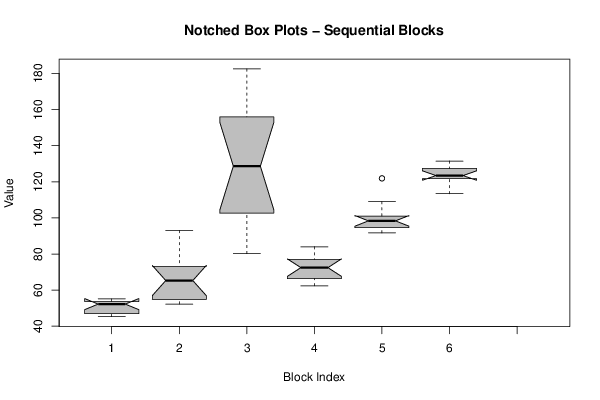PNG link Postscript link PDF link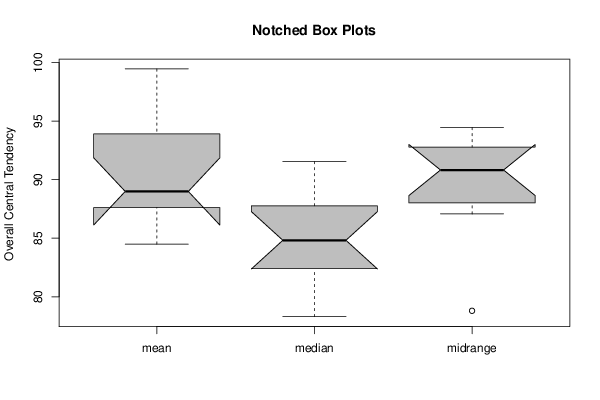PNG link Postscript link PDF link

Parameters (Session):
par1 = 12 ;
Parameters (R input):
par1 = 12 ;
R code (references can be found in the software module):
par1 <- as.numeric(par1)(n <- length(x))(np <- floor(n / par1))arr <- array(NA,dim=c(par1,np+1))darr <- array(NA,dim=c(par1,np+1))ari <- array(0,dim=par1)dx <- diff(x)j <- 0for (i in 1:n){j = j + 1ari[j] = ari[j] + 1arr[j,ari[j]] <- x[i]darr[j,ari[j]] <- dx[i]if (j == par1) j = 0}ariarrdarrarr.mean <- array(NA,dim=par1)arr.median <- array(NA,dim=par1)arr.midrange <- array(NA,dim=par1)for (j in 1:par1){arr.mean[j] <- mean(arr[j,],na.rm=TRUE)arr.median[j] <- median(arr[j,],na.rm=TRUE)arr.midrange[j] <- (quantile(arr[j,],0.75,na.rm=TRUE) + quantile(arr[j,],0.25,na.rm=TRUE)) / 2}overall.mean <- mean(x)overall.median <- median(x)overall.midrange <- (quantile(x,0.75) + quantile(x,0.25)) / 2bitmap(file='plot1.png')plot(arr.mean,type='b',ylab='mean',main='Mean Plot',xlab='Periodic Index')mtext(paste('#blocks = ',np))abline(overall.mean,0)dev.off()bitmap(file='plot2.png')plot(arr.median,type='b',ylab='median',main='Median Plot',xlab='Periodic Index')mtext(paste('#blocks = ',np))abline(overall.median,0)dev.off()bitmap(file='plot3.png')plot(arr.midrange,type='b',ylab='midrange',main='Midrange Plot',xlab='Periodic Index')mtext(paste('#blocks = ',np))abline(overall.midrange,0)dev.off()bitmap(file='plot4.png')z <- data.frame(t(arr))names(z) <- c(1:par1)(boxplot(z,notch=TRUE,col='grey',xlab='Periodic Index',ylab='Value',main='Notched Box Plots - Periodic Subseries'))dev.off()bitmap(file='plot4b.png')z <- data.frame(t(darr))names(z) <- c(1:par1)(boxplot(z,notch=TRUE,col='grey',xlab='Periodic Index',ylab='Value',main='Notched Box Plots - Differenced Periodic Subseries'))dev.off()bitmap(file='plot5.png')z <- data.frame(arr)names(z) <- c(1:np)(boxplot(z,notch=TRUE,col='grey',xlab='Block Index',ylab='Value',main='Notched Box Plots - Sequential Blocks'))dev.off()bitmap(file='plot6.png')z <- data.frame(cbind(arr.mean,arr.median,arr.midrange))names(z) <- list('mean','median','midrange')(boxplot(z,notch=TRUE,col='grey',ylab='Overall Central Tendency',main='Notched Box Plots'))dev.off()